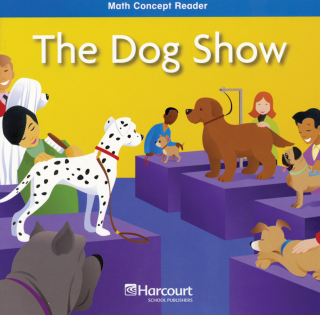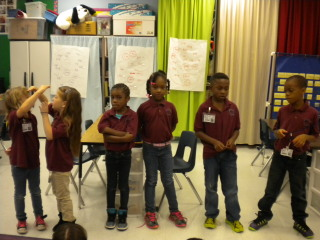# Order Length

28 teachers like this lesson
Print Lesson

## Objective

SWBAT order three objects by length using the terms shortest and longest.

#### Big Idea

The long and the short of it….. In this introductory lesson to ordering lengths, students will be able to compare objects by length using the vocabulary longest and shortest.

## Activating Strategy

10 minutes

To start this lesson, I like to read the following book:  “The Dog Show”.This book demonstrates how to measure dogs by using nonstandard measurements.  It gives the students the idea of longer, taller, and shorter using real world objects.

If this book isn't available, there are many other books about measuring that will get the students engaged and excited about the lesson.  The idea is to get students to apply measuring to real world applications and get their minds thinking about what they already know about measuring.

After reading the book, I randomly call on three or four students and have them line themselves up from shortest to tallest.  I don’t guide them; I allow them to discover how to determine who is the tallest and who is the shortest.  I continue to call up a few students at a time and have them join in on the line.  After all students are lined up, I ask how they decided who was taller and who was shorter.The standard MD.A.1 calls for students to order three objects by length.  In this lesson students will put objects in order by length and draw items in order by length.

## Teaching Strategies

15 minutes

To start the lesson, I show the students a straw and a key.  I have them to draw a picture of something in the classroom that is longer than the straw, and shorter than the key.  I then ask:

• How can you tell if something is longer than the straw? (I can line it up with the straw to see which one ends first.  The one that ends first is the shortest.)
• How can you tell if an object is shorter than the key? ( I can line it up with the key to see which one ends first.)I then have each student get 3 crayons.  I have them line the crayons up from shortest to longest.  I have them turn to their shoulder partner and explain their rationale for lining them up that way.  The idea of doing this is to get them to use the mathematical vocabulary of longest and shortest, along with explaining that they need to line them up so that they start at the same point.  This is in direct alignment with MP6 – attending to precision.  Allowing students to develop their mathematical communication skills while using clear and precise language in their discussions with others as they explain their own reasoning will help them in attending to precision.

## Independent Practice

30 minutes

The independent practice portion of this lesson is designed to further develop their mathematical skills in using the vocabulary longest and shortest, while modeling how to draw items that are longer and shorter.  I use the Order Length worksheet for the independent practice portion of the lesson.

To assist those struggling students, I model using connecting cubes.  For example, I would have the students make a model of 12 cubes, 5 cubes, and 9 cubes.  Discuss how they cube trains are different lengths, and that we can compare them to find out which one is longest and which one is shortest.  Model how to line the ends of the cube trains up and ask:

• Which cube train is the longest? (the 12 cube train)
• Which cube train is the shortest? (the 5 cube train)

I then repeat the activity with three other cube trains of different lengths.

## Closing/Summarizing

5 minutes

To close out the lesson, I like to hand out random sized pieces of yarn – one piece per student.  The students then mix, pair, share and compare their yarn with their partner and discuss which is the longest and which is the shortest.